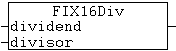# FIX16DivThis function divides two signed 16-bit fixed-point numbers. The numbers do not have to have the same resolution (number of decimal places). The resolution of the number with the higher number of decimal places is reduced before the division. i.e. the decimal places of the number with the higher resolution are truncated. The result of the division is a signed 16-bit fixed-point number.

## VAR_INPUT

``VAR_INPUT    dividend : T_FIX16;    divisor  : T_FIX16;END_VAR``

dividend: Number that is divided (type: T_FIX16).

divisor: Number used for the division (type: T_FIX16).

Example:

``PROGRAM FIXDIVVAR    a, b   : T_FIX16;    result : LREAL;END_VAR``
``a := LREAL_TO_FIX16( -22.5, 8 );b := LREAL_TO_FIX16( 10.0, 8 );result := FIX16_TO_LREAL( FIX16Div( a, b ) ); (* The result is: -2.25 *)``

## Requirements

Development environment

Target platform

PLC libraries to be integrated (category group)

TwinCAT v3.1.0

PC or CX (x86, x64, ARM)

Tc2_Utilities (System)